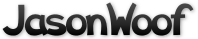Got questions, comments, patches, etc.? Contact Jason Woofenden
 author Joshua Grams Sun, 26 Mar 2006 01:56:24 +0000 (01:56 +0000) committer Joshua Grams Sun, 26 Mar 2006 01:56:24 +0000 (01:56 +0000)
 main.c patch | blob | history

diff --git a/main.c b/main.c
index 94c8e9a..927d2bb 100644 (file)
--- a/main.c
+++ b/main.c
@@ -99,10 +99,20 @@ enum states {
enum states state = TITLE_PAGE;
float state_timeout = 600.0;

+char *space_msgs = {
+       "Press SPACE to start a new game",
+       "Press SPACE for easy game",
+       "Press SPACE for normal game"
+};
+
+char *other_msgs = {
+       "Press 'e' for easy game",
+       "Press 'n' for normal game"
+};
#define NSEQUENCE 3
char *sequence[] = {
"Press SPACE for normal game",
-       "Or 'e' for easy game",
+       "Press 'e' for easy game",
"http://jasonwoof.org/vor"
};

@@ -448,19 +458,19 @@ draw_game_over(void)
if(new_high_score(score)) {
text0 = "New High Score!";
text1 = "Press SPACE to continue";
-       } else if(opt_gamespeed == EASY_GAMESPEED) {
-               text0 = "Press SPACE to start a new game";
-               text1 = "Press 'e' to start an easy game";
+       } else if(initial_rocks == EASY_I_ROCKS) {
+               text0 = space_msgs; sequence = text0;
+               text1 = other_msgs; sequence = text1;
} else {
-               text0 = "Press SPACE to start an easy game";
-               text1 = "Press 'n' to start a normal game";
+               text0 = space_msgs; sequence = space_msgs;
+               text1 = other_msgs; sequence = text1;
}

-       x = (XSIZE-SFont_TextWidth(g_font,text0))/2 + cos(fadetimer/4.5)*10;
-       SFont_Write(surf_screen,g_font,x,YSIZE-100 + cos(fadetimer/3)*5,text0);
+       x = (XSIZE-SFont_TextWidth(g_font,text0))/2 + cos(fadetimer/9)*10;
+       SFont_Write(surf_screen,g_font,x,YSIZE-100 + cos(fadetimer/6)*5,text0);

-       x = (XSIZE-SFont_TextWidth(g_font,text1))/2 + sin(fadetimer/4.5)*10;
-       SFont_Write(surf_screen,g_font,x,YSIZE-50 + sin(fadetimer/2)*5,text1);
+       x = (XSIZE-SFont_TextWidth(g_font,text1))/2 + sin(fadetimer/9)*10;
+       SFont_Write(surf_screen,g_font,x,YSIZE-50 + sin(fadetimer/4)*5,text1);
}

void
@@ -487,7 +497,7 @@ draw_title_page(void)
SDL_SetAlpha(surf_b_rockdodger, SDL_SRCALPHA, (int)(200 + 55*sin(fadetimer-2.0)));
SDL_BlitSurface(surf_b_rockdodger,NULL,surf_screen,&dest);

-       text = sequence[(int)(fadetimer/40)%NSEQUENCE];
+       text = sequence[(int)(fadetimer/35)%NSEQUENCE];
x = (XSIZE-SFont_TextWidth(g_font,text))/2 + cos(fadetimer/4.5)*10;
SFont_Write(surf_screen,g_font,x,YSIZE-100 + cos(fadetimer/3)*5,text);

@@ -605,7 +615,8 @@ gameloop() {
break;
case HIGH_SCORE_DISPLAY:
state = TITLE_PAGE;
-                                               state_timeout = 500.0;
+                                               state_timeout = 600.0;
+                                               fadetimer = 0.0;
break;
case HIGH_SCORE_ENTRY:
break;
@@ -698,7 +709,7 @@ gameloop() {
if(state == GAME_OVER && new_high_score(score))
init_score_entry();
else {
-                                       if(keystate[SDLK_n] || (keystate[SDLK_SPACE] && !initial_rocks)) {
+                                       if((keystate[SDLK_SPACE] && !initial_rocks) || keystate[SDLK_n]) {
initial_rocks = NORMAL_I_ROCKS;
final_rocks = NORMAL_F_ROCKS;
if(opt_gamespeed == EASY_GAMESPEED)